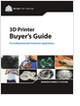Formula HomeHeat TransferOverviewConduction1-D ConductionElectrical AnalogySymbol DefinitionConvectionForced ConvectionFree ConvectionRadiationGlossaryApplicationsLam. Flow Over PlateTwo Body RadiationResourcesBibliography
Selecting the Right 3D Printer

Discover how to choose the right 3D printer for your needs and the key performance attributes to consider.

Essentials of Manufacturing

Information, coverage of important developments and expert commentary in manufacturing.

Salary Expectation

8 things to know about the interview question "What's your salary expectation"?

Learn the best principles to negotiate the salary you deserve!

more free publicationsFor problems where the temperature variation is only 1-dimensional (say, along the x-coordinate direction), Fourier's Law of heat conduction simplies to the scalar equations,where the heat flux q depends on a given temperature profile T and thermal conductivity k. The minus sign ensures that heat flows down the temperature gradient. In the above equation on the right,represents the heat flow through a defined cross-sectional area A, measured in watts,Integrating the 1D heat flow equation through a material's thickness Dx gives,where T1 and T2 are the temperatures at the two boundaries.
The R-Value in InsulationIn general terms, heat transfer is quantified by Newton's Law of Cooling,where h is the heat transfer coefficient. For conduction, h is a function of the thermal conductivity and the material thickness,In words, h represents the heat flow per unit area per unit temperature difference. The larger h is, the larger the heat transfer Q. The inverse of h is commonly defined as the R-value,The R-value is used to describe the effectiveness of insulations, since as the inverse of h, it represents the resistance to heat flow. The larger the R, the less the heat flow. R is often expressed in imperial units when listed in tables. Conversion to SI-units is provided in the Units Section. To convert R into a thermal conductivity k, we must divide the thickness of the insulation by the R value (or just solve for k from the above equation),GlossarySelecting the Right 3D Printer

Discover how to choose the right 3D printer for your needs and the key performance attributes to consider.Essentials of Manufacturing

Information, coverage of important developments and expert commentary in manufacturing.Salary Expectation

8 things to know about the interview question "What's your salary expectation"?